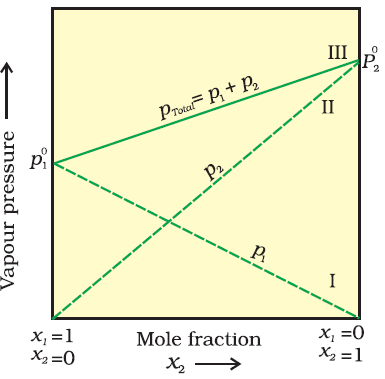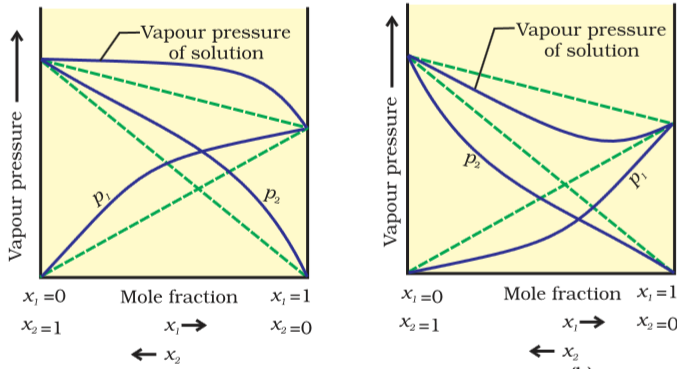The Learning Point‎ > ‎Chemistry‎ > ‎

### A Tutorial on Liquid Solutions: Notes, Figures and Problems with Solutions

Target Audience: These notes on Atomic Structure are meant for college freshmen, or high school students in Grades 11 or 12.

They might be of use to Indian students preparing for the ISC or CBSE Class 11 and 12 Examinations, IIT JEE (main and advanced), AIEEE; students from across the world preparing for their A Level Examinations, IB (International Baccalaureate) or AP Chemistry.

This compilation of notes has been prepared by Rahul Sharma of IIT Kanpur.

## Complete Tutorial with Problems, Figures and Solutions :

### A Summarization and Recap of the (Above) Tutorial on Liquid Solutions

Solutions: - Solutions are homogeneous mixtures of two or more than two components. By homogenous mixture we mean that its composition and properties are uniform throughout the mixture.

Solvent:-The component of the solution having the largest quantity is called solvent.

Solute:-One or more components present in the solution other than the solvent is called solute.

 Type of solution Solvent Solute Common Examples Gaseous solutions Gas Gas Mixture of oxygen and nitrogen gases Liquid Gas Chloroform mixed with nitrogen gas Solid Gas Camphor in nitrogen gas Liquid solutions Gas Liquid Oxygen dissolved in water Liquid Liquid Ethanol dissolved in water Solid Liquid Glucose dissolved in water Solid solutions Gas Solid Solution of hydrogen in palladium Liquid Solid Amalgam of mercury with sodium Solid Solid Copper dissolved in gold

### Expressing concentration of solution:-

There are several ways by which we can describe the concentration of the solution quantitatively.

1. Mass percentage (w/w)

1. Volume percentage (v/v)

1. Mass by volume percentage (w/v)

2. Parts per million

3. Mole fraction

1. Molarity

1. Molality

### Solubility

Solubility of a substance is its maximum amount that can be dissolved in a specified amount of solvent.

### Solubility of solid in liquid

A solution in which no more solute can be dissolved at the same temperature and pressure is called a saturated solution.

The solubility of a solid in a liquid is significantly affected by temperature changes. This, being dynamic equilibrium, must follow Le Chatelier’s Principle. In general, if in a nearly saturated solution, the dissolution process is endothermic (Δsol H < 0), the solubility should increase with rise in temperature and if it is exothermic (Δsol H > 0) the solubility should decrease.

Pressure does not have any significant effect on solubility of solids in liquids. It is so because solids and liquids are highly incompressible and practically remain unaffected by changes in pressure.

### Solubility of gases in liquid:-

Solubility of gases in liquids is greatly affected by pressure and temperature. The solubility of gases increase with increase of pressure.

### Henry’s law

the partial pressure of the gas in vapour phase (p) is proportional to the mole fraction of the gas (x) in the solution.

Solubility of gases in liquids decreases with rise in temperature. When dissolved, the gas molecules are present in liquid phase and the process of dissolution can be considered similar to condensation and heat is evolved in this process. Thus this is an exothermic process.

When taken in a closed vessel, both the components would evaporate and eventually equilibrium would be established between vapour phase and the liquid phase.

### Raoult’s law

A French chemist Raoult states that for a solution of volatile liquids, the partial vapour pressure of each component in the solution is directly proportional to its mole fraction.

1. Total vapour pressure over the solution can be related to the mole fraction of any one component.

2. Total vapour pressure over the solution varies linearly with the mole fraction of component 2.

3. Depending on the vapour pressures of the pure components 1 and 2, total vapour pressure over the solution decreases or increases with the increase of the mole fraction of component 1### Vapour pressure of solutions of solid in liquid

If a non-volatile solute is added to a solvent to give a solution, the vapour pressure of the solution is solely from the solvent alone. This vapour pressure of the solution at a given temperature is found to be lower than the vapour pressure of the pure solvent at the same temperature. In the solution, the surface has both solute and solvent molecules; thereby the fraction of the surface covered by the solvent molecules gets reduced. Consequently, the number of solvent molecules escaping from the surface is correspondingly reduced, thus, the vapour pressure is also reduced.

The decrease in the vapour pressure of solvent depends on the quantity of non-volatile solute present in the solution, irrespective of its nature.

### Ideal solution

The solutions which obey Raoult’s law over the entire range of concentration are known as ideal solutions.

No heat is absorbed or evolved when the components are mixed. Also, the volume of solution would be equal to the sum of volumes of the two components.

∆mixH=0 and ∆mixV=0

In pure components, the intermolecular attractive interactions will be of types A-A and B-B, whereas in the binary solutions in addition to these two interactions, A-B type of interactions will also be present. If the intermolecular attractive forces between the A-A and B-B are nearly equal to those between A-B, this leads to the formation of ideal solution.

Ex. N-hexane and n-heptane

Bromoethane and chloroethane

### Non-ideal solution

When a solution does not obey Raoult’s law over the entire range of concentration, then it is called non-ideal solution. If the vapour pressure is higher, the solution exhibits positive deviation and if it is lower, it exhibits negative deviation from Raoult’s law.

In case of positive deviation from Raoult’s law, A-B interactions are weaker than those between A-A or B-B, i.e., in this case the intermolecular attractive forces between the solute-solvent molecules are weaker than those between the solute-solute and solvent-solvent molecules. This means that in such solutions, molecules of A (or B) will find it easier to escape than in pure state. This will increase the vapour pressure and thus results in positive deviation.

In case of negative deviations from Raoult’s law, the intermolecular attractive forces between A-A and B-B are weaker than those between A-B and lead to decrease in vapour pressure resulting in negative deviations. A mixture of chloroform and acetone forms a solution with negative deviation from Raoult’s law. This is because chloroform molecule is able to form hydrogen bond with acetone.Positive deviation                                  Negative deviation

All those properties depend on the number of solute particles irrespective of their nature relative to the total number of particles present in the solution, are called colligative properties.

Relative lowering of vapour pressure

The vapour pressure of a solvent in solution is less than that of the pure solvent. Raoult established that the lowering of vapour pressure depends only on the concentration of the solute particles and it is independent of their identity.

Elevation in boiling point

As we know that the vapour pressure of a solvent decreases when non-volatile solute is added to it. Boiling point is the temperature at which the vapour pressure of the liquid is equal to the atmospheric pressure. And vapour pressure of solution increases as the temperature is increased.

### Depression in freezing point

The freezing point of a substance may be defined as the temperature at which the vapour pressure of the substance in its liquid phase is equal to its vapour pressure in the solid phase. A solution will freeze when its vapour pressure equals the vapour pressure of the pure solid solvent.

The proportionality constant, Kf, which depends on the nature of the solvent is known as Freezing Point Depression Constant or Molal

Depression Constant or Cryoscopic Constant.

### Osmosis and osmotic pressure

If semi permeable membrane is placed between the solvent and solution the solvent molecules will flow through the membrane from pure solvent to the solution. This process of flow of the solvent is called osmosis. osmotic pressure of the solution.

The important point to be kept in mind is that solvent molecules always flow from lower concentration to higher concentration of solution.

Osmotic pressure is proportional to the molarity, C of the solution at a given temperature T. π=CRT

Two solutions having same osmotic pressure at a given temperature are called isotonic solutions.

### Reverse osmosis(RO)

The direction of osmosis can be reversed if a pressure larger than the osmotic pressure is applied to the solution side. That is, now the pure solvent flows out of the solution through the semi permeable membrane. This phenomenon is called reverse osmosis and is of great practical utility.

### Abnormal masses

Van’t Hoff introduced a factor i, known as the Van’t Hoff factor, to account for the extent of dissociation or association.

A Quick Look at some of the problems we solved in the (above) tutorial:

Example 1: Which of the following liquid pairs shows a positive deviation from Raoult’s law?

Example 2: Which one of the following is non-ideal solution

Example 3: A non ideal solution was prepared by mixing 30 ml chloroform and 50 ml acetone. The volume of mixture will be

Example 4: Which pair from the following will not form an ideal solution

Example 5: An ideal solution is that which

Example 6: A 0.020 m solution of each of the following compounds is prepared. Which solution would you expect to freeze at−0.149°C( Kf water = 1.86° C) ?

Example 7: . Statement – 1: For liquids of isomeric compounds with same kind of association, higher the b.p. higher is the molal elevation constant (Kb).

Example 8-Calculate the mole fraction of ethylene glycol (C2H6O2) in a solution containing 20% of C2H6O2 by mass.

Example 9:- If N2 gas is bubbled through water at 293 K, how many millimoles of N2 gas would dissolve in 1 litre of water. Assume that N2 exerts a partial pressure of 0.987 bar. Given that Henry’s law constant for N2 at 293 K is 76.48 kbar.

Example 10:- The vapour pressure of pure benzene at a certain temperature is 0.850 bar. A non-volatile, non-electrolyte solid weighing 0.5 g when added to 39.0 g of benzene (molar mass 78 g mol-1). Vapour pressure of the solution, then, is 0.845 bar. What is the molar mass of the solid substance?

Example 11:- 45 g of ethylene glycol (C2H6O2) is mixed with 600 g of water. Calculate

(a) the freezing point depression and (b) the freezing point of the solution.

Example 12:- 0.6 mL of acetic acid (CH3COOH), having density 1.06 g mL–1, is dissolved in 1 litre of water. The depression in freezing point observed for this strength of acid was 0.0205°C. Calculate the van’t Hoff factor and the dissociation constant of acid.